# customAntennaGeometry

Create antenna represented by 2-D custom geometry

## Description

The `customAntennaGeometry` object is an antenna represented by a 2-D custom geometry on the xy-plane. Using `customAntennaGeometry`, you can import a planar mesh, define the feed for this mesh to create an antenna, analyze the antenna, and use it in finite or infinite arrays. The image shown is a custom slot antenna.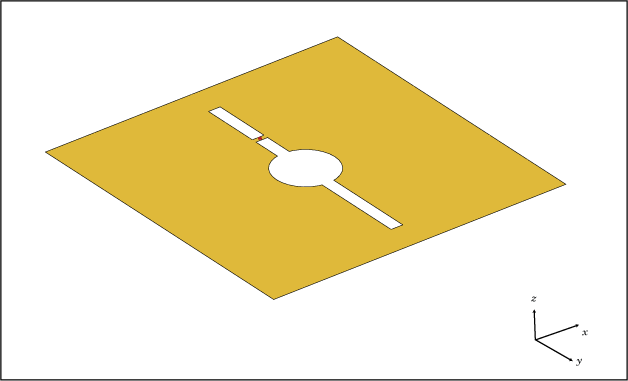## Creation

### Syntax

``ca = customAntennaGeometry``
``ca = customAntennaGeometry(Name=Value)``

### Description

example

``` `ca = customAntennaGeometry` creates a 2-D antenna represented by a custom geometry, based on the specified boundary.```

example

``` `ca = customAntennaGeometry(Name=Value)` creates a 2-D planar antenna geometry, with additional Properties specified by one or more name-value arguments. `Name` is the property name and `Value` is the corresponding value. You can specify several name-value arguments in any order as `Name1=Value1`, `...`, `NameN=ValueN`. Properties not specified retain their default values.```

## Properties

expand all

Boundary information in Cartesian coordinates, specified as a cell array in meters.

Data Types: `double`

Boolean operation performed on the boundary list, specified as a character vector.

Example: `'P1-P2'`

Data Types: `char`

Antenna feed location in Cartesian coordinates, specified as a three-element vector. The three-element vector is the x, y, and z coordinates respectively.

Example: `[0 0.2 0]`

Data Types: `double`

Width of feed section, specified as a scalar in meters.

Example: `0.05`

Data Types: `double`

Type of the metal used as a conductor, specified in the metal material object. You can choose any metal from the `MetalCatalog` or specify a metal of your choice. For more information, see `metal`. For more information on metal conductor meshing, see Meshing.

Example: `metal("Copper")`

Lumped elements added to the antenna feed, specified a `lumpedElement` object.

Example: `lumpedElement(Impedance=75)`

Tilt angle of the antenna in degrees, specified as a scalar or vector. For more information, see Rotate Antennas and Arrays.

Example: `90`

Example: `Tilt=[90 90]`,`TiltAxis=[0 1 0;0 1 1]` tilts the antenna at 90 degrees about the two axes defined by the vectors.

Data Types: `double`

Tilt axis of the antenna, specified as one of these values:

• Three-element vector of Cartesian coordinates in meters. In this case, each coordinate in the vector starts at the origin and lies along the specified points on the x-, y-, and z-axes.

• Two points in space, specified as a 2-by-3 matrix corresponding to two three-element vectors of Cartesian coordinates. In this case, the antenna rotates around the line joining the two points.

• `"x"`, `"y"`, or `"z"` to describe a rotation about the x-, y-, or z-axis, respectively.

Example: `[0 1 0]`

Example: `[0 0 0;0 1 0]`

Example: `"Z"`

Data Types: `double` | `string`

## Object Functions

 `show` Display antenna, array structures, shapes, or platform `info` Display information about antenna or array `axialRatio` Axial ratio of antenna `beamwidth` Beamwidth of antenna `charge` Charge distribution on antenna or array surface `current` Current distribution on antenna or array surface `efficiency` Radiation efficiency of antenna `EHfields` Electric and magnetic fields of antennas or embedded electric and magnetic fields of antenna element in arrays `impedance` Input impedance of antenna or scan impedance of array `mesh` Mesh properties of metal, dielectric antenna, or array structure `meshconfig` Change meshing mode of antenna, array, custom antenna, custom array, or custom geometry `pattern` Plot radiation pattern and phase of antenna or array or embedded pattern of antenna element in array `patternAzimuth` Azimuth plane radiation pattern of antenna or array `patternElevation` Elevation plane radiation pattern of antenna or array `rcs` Calculate and plot radar cross section (RCS) of platform, antenna, or array `returnLoss` Return loss of antenna or scan return loss of array `sparameters` Calculate S-parameters for antennas and antenna arrays `vswr` Voltage standing wave ratio (VSWR) of antenna or array element

## Examples

collapse all

Create a custom dipole antenna and view it.

`ca = customAntennaGeometry`
```ca = customAntennaGeometry with properties: Boundary: {[4x3 double]} Operation: 'P1' FeedLocation: [0 0 0] FeedWidth: 0.0200 Conductor: [1x1 metal] Tilt: 0 TiltAxis: [1 0 0] Load: [1x1 lumpedElement] ```
`show(ca)`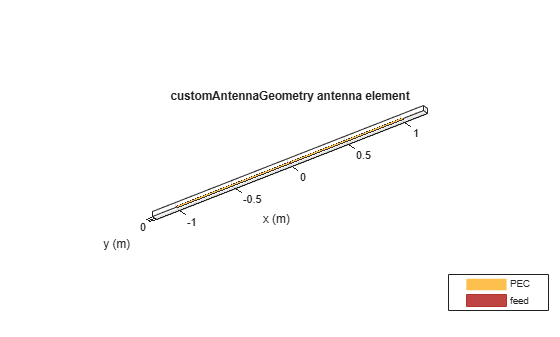Create a custom slot antenna using three rectangles and a circle.

Create three rectangles of dimensions 0.5 m x 0.5 m, 0.02 m x 0.4 m and 0.03 m x 0.008 m and get the coordinates of their vertices.

```pr = antenna.Rectangle(Length=0.5,Width=0.5); boundary_1 = getShapeVertices(pr); pr1 = antenna.Rectangle(Length=0.02,Width=0.4); boundary_2 = getShapeVertices(pr1); pr2 = antenna.Rectangle(Length=0.03,Width=0.008);```

Create a circle of radius 0.05 m.

```ph = antenna.Circle(Radius=0.05); boundary_4 = getShapeVertices(ph);```

Translate the third rectangle to the X-Y plane using the coordinates [0 0.1 0]. Get coordinates of its vertices.

```pf = translate(pr2,[0 0.1 0]); boundary_3 = getShapeVertices(pf);```

Use customAntennaGeometry object to create a custom slot antenna shape. Specify the shape boundaries and add/subtract operations to get the desired shape. Set the feed location and feed width. View the custom slot antenna.

```c = customAntennaGeometry; c.Boundary = {boundary_1, boundary_2, boundary_3, boundary_4}; c.Operation = 'P1-P2-P4+P3'; c.FeedWidth = 0.01; c.FeedLocation = [0 0.1 0]; figure show(c)```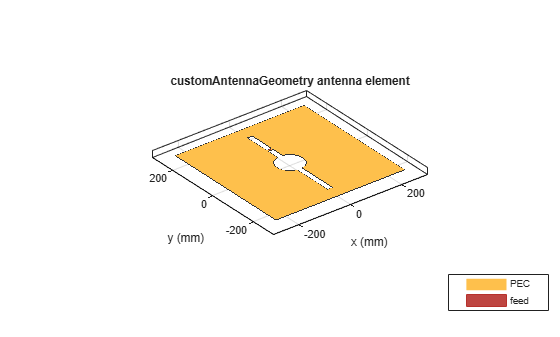Analyze the impedance of the antenna in 300 MHz to 800 MHz frequency range.

```figure impedance(c,linspace(300e6,800e6,51));```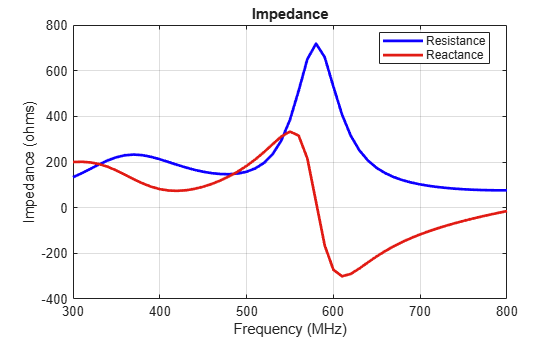Analyze the current distribution of the antenna at 575 MHz.

```figure current(c,575e6)```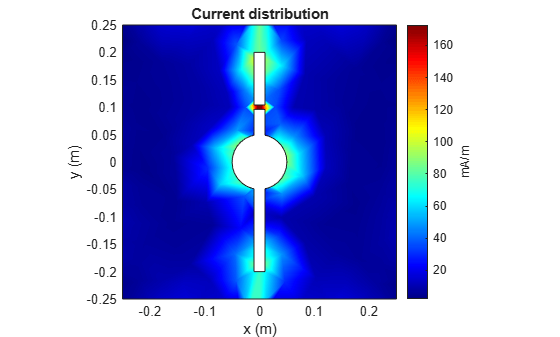Plot the radiation pattern of the antenna at 575 MHz.

```figure pattern(c,575e6)```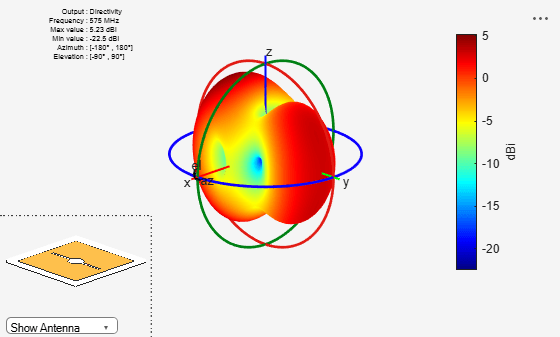Balanis, C. A. Antenna Theory. Analysis and Design. 3rd Ed. Hoboken, NJ: John Wiley & Sons, 2005.